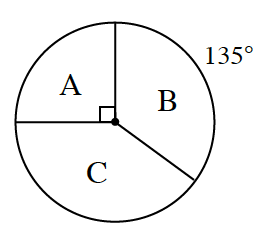### Home > CCG > Chapter 10 > Lesson 10.2.2 > Problem10-86

10-86.

The spinner below has three regions: $\text{A, B, and C}$. If it is spun $80$ times, how many times would you expect each region to result? Show your work. Homework Help ✎• What fraction of this circle is each of the regions? Multiply the fraction by the number of spins.